# Three-node Example to Compute iRAM (impulse response analysis metric)

### Overview

We created an R package named “pompom” (person-oriented modeling and perturbation on the model), and we will use the functions in “pompom” to compute iRAM (impulse response analysis metric) in this tutorial.

iRAM is built upon a hybrid method that combines intraindividual variability methods and network analysis methods in order to model individuals as high-dimensional dynamic systems. This hybrid method is designed and tested to quantify the extent of interaction in a high-dimensional multivariate system, and applicable on experience sampling data.

Note: please install the package “pompom”, before running the code in this tutorial.

This turorial corresponds to the following paper (under review): Yang et al. Impulse Response Analysis Metric (iRAM): Merging Intraindividual Variability, Network Analysis and Experience Sampling

### Outline of Tutorial

1. Prepare simulation of time-series data
2. Apply Intraindividual Variability Method (unified Structural SEM, uSEM) on multivariate time-series data
3. Apply a network analysis method (also called impulse reponse analysis) on person-specific network
4. Calculate impulse response analysis metric (iRAM)

### Step 1. Prepare simulation of time-series data

library(pompom)
library(ggplot2)
library(qgraph)
require(reshape2)
set.seed(1234)

#### Set up a 3 node model

The 3-node network is a hypothetical example used for the purpose of illustration. As explained in the paper, the variables in time-series data are nodes and the temporal relations are edges, in the network terminology.

n.obs <- 200 # number of observation
p <- 3 # number of variables
noise.level <- 0.1
epsilon <- cbind(rnorm(n.obs,0, noise.level),
rnorm(n.obs,0, noise.level),
rnorm(n.obs,0, noise.level)) # 3 time-series of noise for 3 variables

true.beta <-  matrix(c(0,0,0,0,0,0,
0,0,0,0,0,0,
0,0,0,0,0,0,
0.2,0,0.25,0,0,0.6,
0,0.3,0,-0.2,0,-0.6,
0,-0.2,0.3,0,0,0),
nrow = 2 * p,
ncol = 2 * p,
byrow = TRUE)

contemporaneous.relations <- matrix(true.beta[(p+1):(2*p),(p+1):(2*p)], nrow = p, ncol = p, byrow = F)
lag.1.relations <- matrix(true.beta[(p+1):(2*p),1:p], nrow = p, ncol = p, byrow = F)

True model is:

true.beta
##      [,1] [,2] [,3] [,4] [,5] [,6]
## [1,]  0.0  0.0 0.00  0.0    0  0.0
## [2,]  0.0  0.0 0.00  0.0    0  0.0
## [3,]  0.0  0.0 0.00  0.0    0  0.0
## [4,]  0.2  0.0 0.25  0.0    0  0.6
## [5,]  0.0  0.3 0.00 -0.2    0 -0.6
## [6,]  0.0 -0.2 0.30  0.0    0  0.0

#### Network graph

To introduce the terminologies of networks, each of the 3 variables is depicted as a node (circle), and the temporal relations among the nodes are depicted as edges (arrows, and the arrow indicate directionality of the temporal relationship), with color indicating sign of relation (green = positive, red = negative), thickness indicating strength of relation, and line shape indicating temporality of the association (dashed = lag-1, solid = contemporaneous). Lag-1 relations mean the temporal relations between variables from measurement t -1 to the measurement t, and contemporaneous relations means the temporal relations between variables within the same measurement.

plot_network_graph(true.beta, p)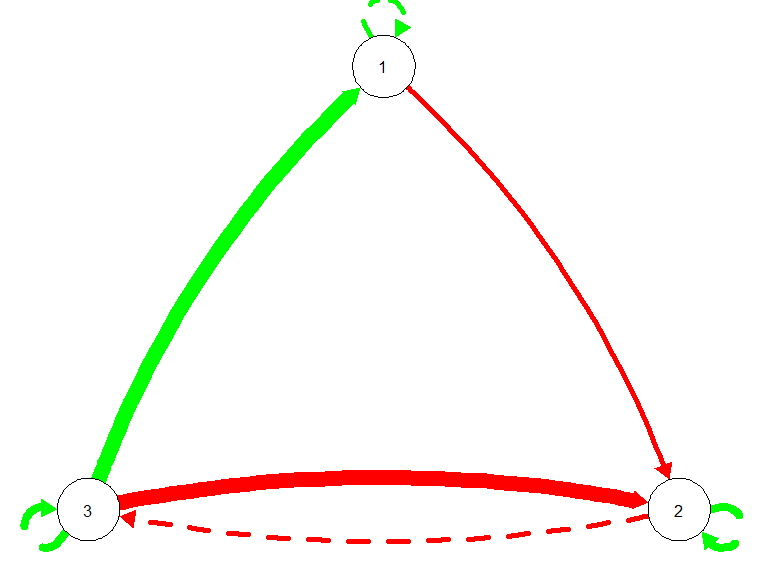## NULL

#### Simulate time-series

Time series was simulated based on the temporal relations and process noise. Our hypohetical 3-node newtork was using simulated data based on a pre-defined temporal relationship matrix and process noise. The temporal relationship is as follows, and process noise is at Mean = 0, SD = .1.

$$\eta(t) = (I-\ A \ )^{-1}\Phi\eta(t-1) + (I-\ A \ )^{-1}\epsilon(t)$$

where $$\ A$$ is the block of contemporaneous relations (southeast block of the beta matrix),

$$\Phi$$ is the block of lag-1 relations (southwest block of the beta matrix),

$$\epsilon$$ is the process noise.

We simulated 200 occasions to represent 200 repeated measurements of the three variables in the real studies.

time.series <- matrix(rep(0, p * n.obs), nrow = n.obs, ncol = p)
time.series[1,] <- rnorm(p,0,1)

row <- p
for (row in 2:n.obs)
{
time.series[row,] <- solve(diag(1,p, p) - contemporaneous.relations) %*%
lag.1.relations %*% time.series[row-1,] +
solve(diag(1,p, p) - contemporaneous.relations) %*% epsilon[row, ]
}
time.series <- data.frame(time.series)
names(time.series) <- c("x", "y", "z")

#### Plot the simulated time-series

time.series$time <- seq(1,length(time.series[,1]),1) time.series.long <- melt(time.series, id="time") ## convert to long format ggplot(data=time.series.long, aes(x=time, y=value, colour=variable)) + geom_line() + facet_wrap( ~ variable , ncol = 1) + scale_y_continuous(breaks = seq(0, 100, by = 50)) + theme( strip.background = element_blank(), panel.background = element_blank(), legend.title = element_blank(), # strip.text.x = element_blank(), # strip.text.y = element_blank(), # axis.text.y = element_text(), # axis.title.y = element_blank() legend.key = element_blank(), legend.position = "none", # legend.title = element_blank(), # panel.background = element_blank(), axis.text.y=element_text(color="black",size=12), axis.text.x=element_text(color="black",size=12), axis.title.y=element_text(color="black",size=12), axis.title.x=element_text(color="black",size=12), axis.line = element_line(color = 'black') )+ ylim(-1,1)+ xlab("Time") + ylab("Value") ## Scale for 'y' is already present. Adding another scale for 'y', which ## will replace the existing scale. ## Warning: Removed 1 rows containing missing values (geom_path).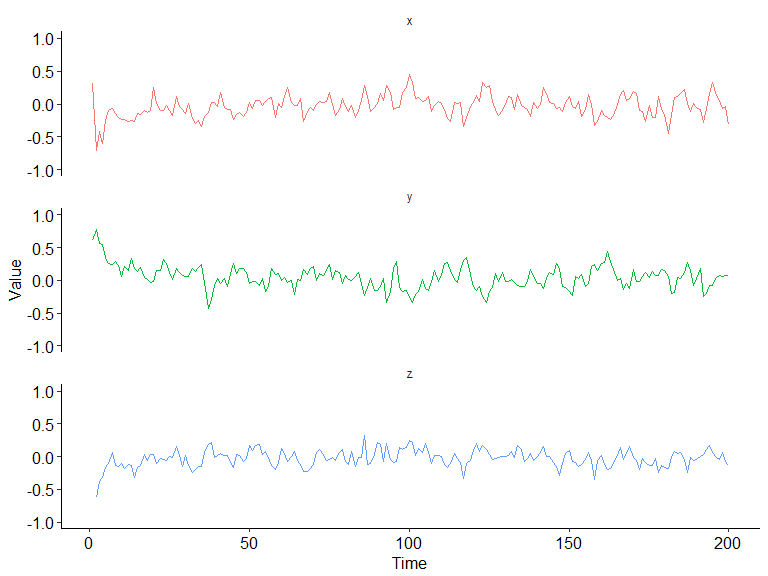time.series$time <- NULL

### Step 2. Apply Intraindividual Variability Method (unified Structural SEM, uSEM) on multivariate time-series data

#### Use uSEM to fit the model and parse parameters.

The model fit summary will give and estimates, which are essential information to conduct network analysis later. The model fit summary also gives the fit statistics.

Parameters of the “uSEM” function:

var.number: number of variables, 13 in this case.

time.series: multivariate time-series data in the long format (every row is a measurement, and every column is a variable). Note: scaling is not absolutely necessary, but it can be helpful when some variables have small variances.

lag.order: number of lags in the uSEM model. Default is 1.

verbose: default at FALSE. If TRUE, it will print the top five largest modification indices in the lavaan model of each iteration.

trim: default at TRUE. If TRUE, it will trim the final model, eliminating all the insignificant temporal relations.

var.number <- p # number of variables
lag.order <- 1 # lag order of the model

model.fit <- uSEM(var.number,
time.series,
lag.order,
verbose = FALSE,
trim = TRUE)

Now the uSEM model result is in the object “model.fit”, including beta matrix, psi matrix, and fit statistics. Next, we need to parse the model.fit object into beta matrix and plot the estimated network graph.

beta.matrix <- parse_beta(var.number = p,
model.fit = model.fit,
lag.order = 1,
matrix = TRUE) # parse temporal relations in matrix format

plot_network_graph(beta.matrix$est, var.number)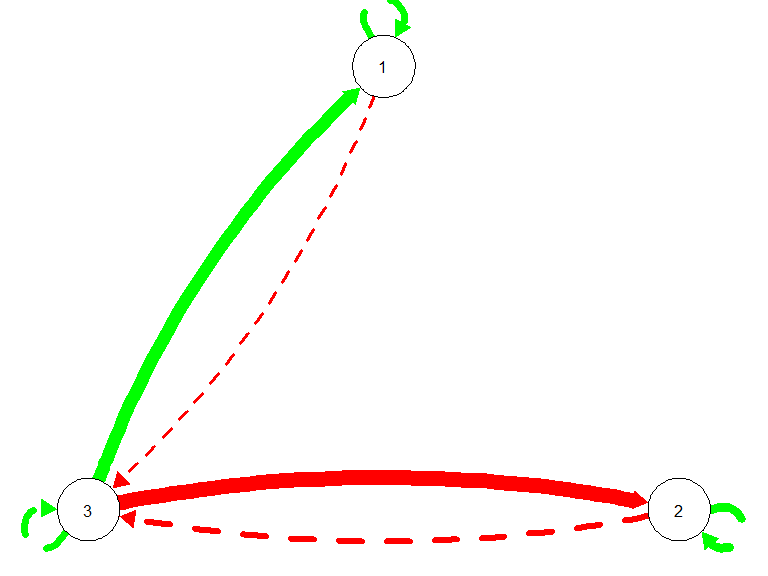## NULL estimated beta0 = beta.matrix$est
##            [,1]       [,2]      [,3] [,4] [,5]       [,6]
## [1,]  0.0000000  0.0000000 0.0000000    0    0  0.0000000
## [2,]  0.0000000  0.0000000 0.0000000    0    0  0.0000000
## [3,]  0.0000000  0.0000000 0.0000000    0    0  0.0000000
## [4,]  0.3066036  0.0000000 0.2527896    0    0  0.5761694
## [5,]  0.0000000  0.4078627 0.0000000    0    0 -0.6707407
## [6,] -0.1091465 -0.2513549 0.3145093    0    0  0.0000000
beta.matrix$se ## [,1] [,2] [,3] [,4] [,5] [,6] ## [1,] 0.00000000 0.00000000 0.00000000 0 0 0.00000000 ## [2,] 0.00000000 0.00000000 0.00000000 0 0 0.00000000 ## [3,] 0.00000000 0.00000000 0.00000000 0 0 0.00000000 ## [4,] 0.04621379 0.00000000 0.05333449 0 0 0.06745900 ## [5,] 0.00000000 0.04745960 0.00000000 0 0 0.06460644 ## [6,] 0.05327602 0.06321407 0.05600953 0 0 0.00000000 The “model_summary” function will return an object that contains beta, psi and fit statistics, shown in the following code chunks. mdl <- model_summary(model.fit, var.number, lag.order) mdl$beta
##            [,1]       [,2]      [,3] [,4] [,5]       [,6]
## [1,]  0.0000000  0.0000000 0.0000000    0    0  0.0000000
## [2,]  0.0000000  0.0000000 0.0000000    0    0  0.0000000
## [3,]  0.0000000  0.0000000 0.0000000    0    0  0.0000000
## [4,]  0.3066036  0.0000000 0.2527896    0    0  0.5761694
## [5,]  0.0000000  0.4078627 0.0000000    0    0 -0.6707407
## [6,] -0.1091465 -0.2513549 0.3145093    0    0  0.0000000
mdl$beta.se ## [,1] [,2] [,3] [,4] [,5] [,6] ## [1,] 0.00000000 0.00000000 0.00000000 0 0 0.00000000 ## [2,] 0.00000000 0.00000000 0.00000000 0 0 0.00000000 ## [3,] 0.00000000 0.00000000 0.00000000 0 0 0.00000000 ## [4,] 0.04621379 0.00000000 0.05333449 0 0 0.06745900 ## [5,] 0.00000000 0.04745960 0.00000000 0 0 0.06460644 ## [6,] 0.05327602 0.06321407 0.05600953 0 0 0.00000000 mdl$psi
##             [,1]        [,2]        [,3]       [,4]       [,5]       [,6]
## [1,]  0.02856458 -0.01829867  0.01245015 0.00000000 0.00000000 0.00000000
## [2,] -0.01829867  0.03239941 -0.02224377 0.00000000 0.00000000 0.00000000
## [3,]  0.01245015 -0.02224377  0.03176525 0.00000000 0.00000000 0.00000000
## [4,]  0.00000000  0.00000000  0.00000000 0.01006503 0.00000000 0.00000000
## [5,]  0.00000000  0.00000000  0.00000000 0.00000000 0.01009431 0.00000000
## [6,]  0.00000000  0.00000000  0.00000000 0.00000000 0.00000000 0.01029627

Model fit

Model fit should obey a 3 out of 4 rule (CFI and TLI should be at least .95, and RMSEA and SRMR should be no greater than .08).

mdl$cfi ## cfi ## 0.9828771 mdl$tli
##       tli
## 0.9743157
mdl$rmsea ## rmsea ## 0.07733488 mdl$srmr
##      srmr
## 0.1207021

### Step 3. Apply a network analysis method (also called impulse reponse analysis) on person-specific network

Conceptually, impulse response analysis is to perturb the system (the whole psychological network we estimated) by one node (or multiple nodes, but since this model is linear, the multiple nodes scenario is additive of the single node impulse. Thus one can conduct impulse response analysis in either way and get equivalent result). After the system receives such perturbation, or impulse, the impulse will reverbate through the network based on the statistical relationship.

For example, one estimated sadness can be predicted by -0.35 happiness + 0.2 anger - 0.6 self-esteem, if happiness or anger/self-esteem received a perturbation, then one can deduct what is the value of sadness for the next step, and we can also deduct value of other nodes in the same way. So one can have a time profile per node after the perturbation. Time profile is the trajectory of a variable after the system received the perturbation.

This method will give an intuitive view of the dynamic behavior of a network, in complimentary of the static newtork configuration (e.g. density, centrality, clustering, etc).

Since uSEM estimated contemporaneous relations as well, we transformed the contemporaneous relations back to a traditional lagged format, so that one can conduct impulse response analysis. Details of mathematical deduction is shown in Gates et al. (2010).

#### Set parameters for impulse response analysis

steps <- 100 # number of steps to generate time profile
replication <- 200 # number of repilcations in bootstrap
threshold <- .01 # setting threshold for approximate asymptote (iRAM calculation)

### Step 4. Calculate impulse response analysis metric (iRAM)

#### Point estimation of iRAM

# ponit estimate of iRAM
point.estimate.iRAM <- iRAM(model.fit,
beta = NULL,
var.number = var.number,
lag.order = lag.order,
threshold = threshold,
boot = FALSE,
replication = replication,
steps= steps)

point.estimate.iRAM$recovery.time  ## [,1] [,2] [,3] ## [1,] 9 9 7 ## [2,] 11 11 10 ## [3,] 11 11 9 # point.estimate.iRAM$time.series.data

plot_time_profile(point.estimate.iRAM$time.series.data, var.number = 3, threshold = threshold, xupper = 50)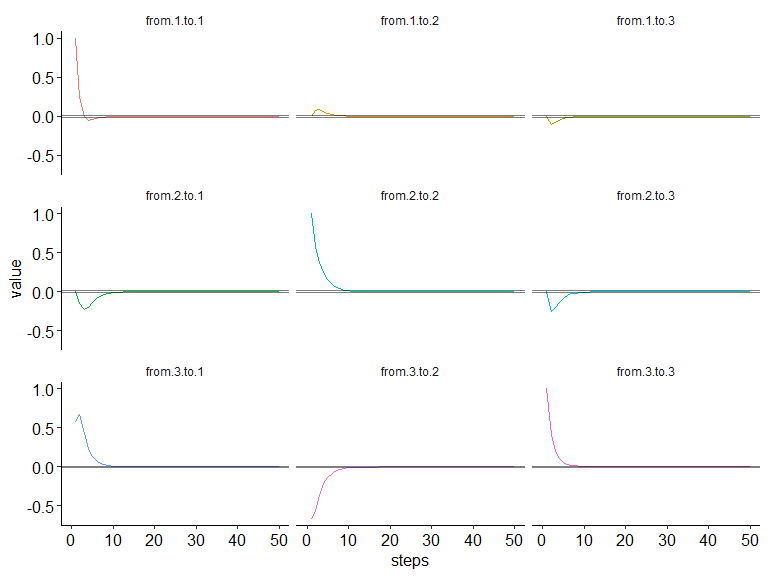## NULL #### Bootstraped iRAM bootstrap.iRAM <- iRAM(model.fit, beta = NULL, var.number = var.number, lag.order = lag.order, threshold = threshold, boot = TRUE, replication = replication, steps= steps ) bootstrap.iRAM$mean
##        [,1]   [,2]  [,3]
## [1,]  8.235  8.340 6.965
## [2,] 11.400 11.575 9.990
## [3,] 10.900 11.085 9.460
bootstrap.iRAM$upper ## [,1] [,2] [,3] ## [1,] 12 13 11 ## [2,] 17 18 17 ## [3,] 18 19 16 bootstrap.iRAM$lower
##      [,1] [,2] [,3]
## [1,]    4    5    4
## [2,]    8    7    6
## [3,]    6    7    5

plot_time_profile(bootstrap.iRAM$time.profile.data, var.number = 3, threshold = threshold, xupper = 25)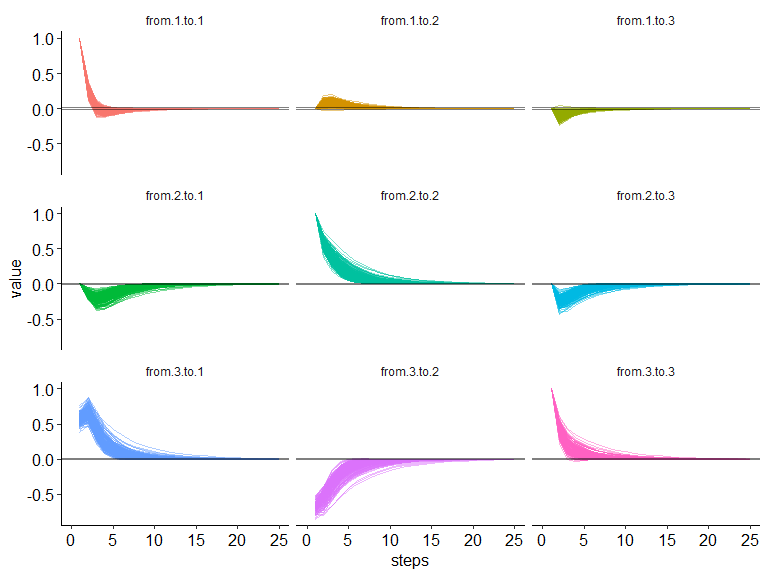## NULL #### Plot boostrapped iRAM distribution plot_iRAM_dist(bootstrap.iRAM$recovery.time.reps)
## stat_bin() using bins = 30. Pick better value with binwidth.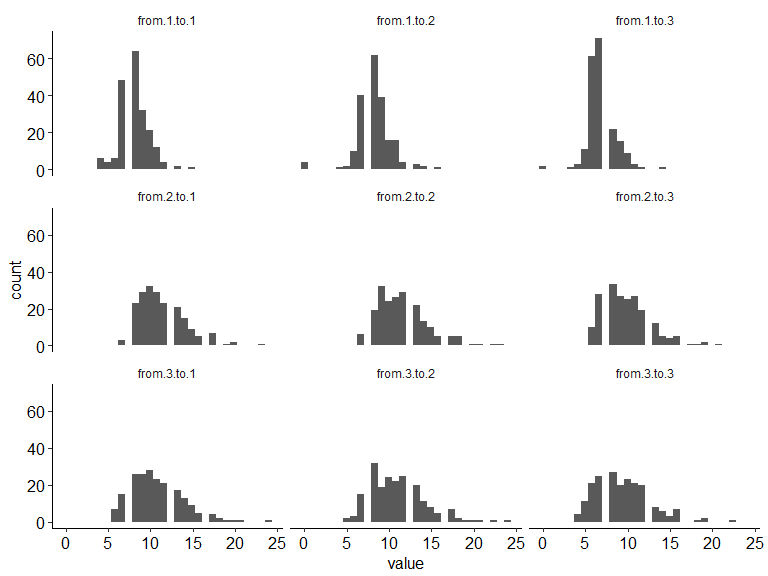## NULL

#### Check accuracy of iRAM estimation

true.iRAM <- iRAM(
model.fit= NULL,
beta = true.beta,
var.number = var.number,
lag.order = lag.order,
threshold = threshold,
boot = FALSE,
replication = replication,
steps= steps)

plot_time_profile(true.iRAM$time.series.data, var.number = 3, threshold = threshold, xupper = 25)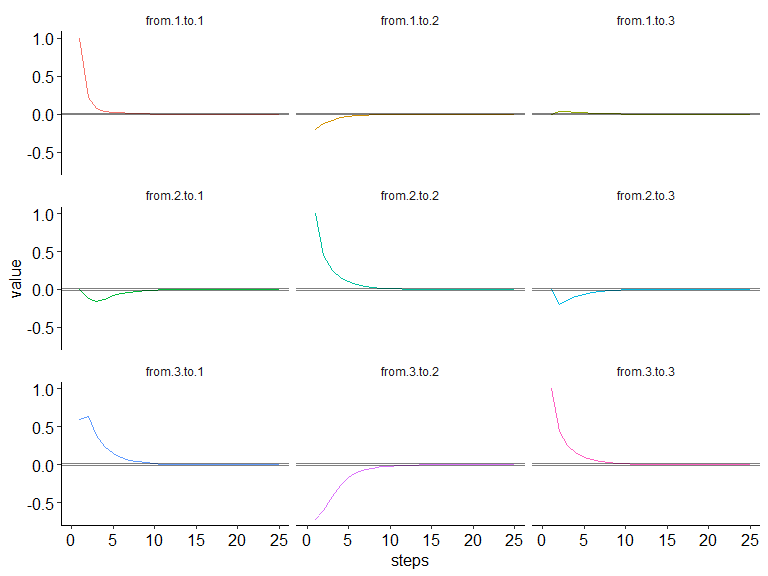## NULL sum.diff <- 0 for (row in 1:nrow(bootstrap.iRAM$recovery.time))
{
sum.diff <- sum.diff + (bootstrap.iRAM$recovery.time.reps[row,] - c(true.iRAM$recovery.time[1,],
true.iRAM$recovery.time[2,], true.iRAM$recovery.time[3,]))^2
}
RMSE <- sqrt(sum.diff/nrow(bootstrap.iRAM$recovery.time)) sum.diff <- 0 for (row in 1:nrow(bootstrap.iRAM$recovery.time))
{
sum.diff <- sum.diff + (bootstrap.iRAM$recovery.time.reps[row,] - c(true.iRAM$recovery.time[1,],
true.iRAM$recovery.time[2,], true.iRAM$recovery.time[3,]))/
(c(true.iRAM$recovery.time[1,], true.iRAM$recovery.time[2,],
true.iRAM$recovery.time[3,])) } RB <- 1/nrow(bootstrap.iRAM$recovery.time) * sum.diff

SD <- NULL
for (col in 1:(var.number^2))
{
SD <- cbind(SD, sd(bootstrap.iRAM$recovery.time.reps[,col])) } #### Summary statistics true.iRAM: iRAM calculated based on true beta matrix. boostrap.iRAM$mean: the iRAM calcualted across bootstrapped replications.

RMSE: root mean squared error of the estimated iRAM compared with true iRAM across bootstrapped replications.

RB: relative bias, mean of summed difference between the estimated iRAM and the true iRAM across bootstrapped replications.

SD: standard deviation of the estimated iRAM across bootstrapped replications.

# metrics
true.iRAM$recovery.time # true value ## [,1] [,2] [,3] ## [1,] 8 8 7 ## [2,] 10 11 9 ## [3,] 11 12 11 bootstrap.iRAM$mean # estimated mean
##        [,1]   [,2]  [,3]
## [1,]  8.235  8.340 6.965
## [2,] 11.400 11.575 9.990
## [3,] 10.900 11.085 9.460
RMSE
##  1.719011 2.111871 1.629417 3.078961 3.007491 2.944486 3.091925 3.378609
##  3.528456
RB
##   0.029375000  0.042500000 -0.005000000  0.140000000  0.052272727
##   0.110000000 -0.009090909 -0.076250000 -0.140000000
SD 
##          [,1]     [,2]     [,3]     [,4]    [,5]     [,6]     [,7]
## [1,] 1.707146 2.089553 1.633129 2.749143 2.95942 2.780026 3.098062
##          [,8]     [,9]
## [1,] 3.260511 3.182616

#### Integrated form of impulse respnose analysis

Sometimes the raw time-series data is non-stationary (e.g., the absolute value of auto-regression is close to or above 1), and one way to model the dynamics is to take the difference score of the time-series and then model the difference scores.

In this case, the temporal relations are describing how the difference score are predicting one another, so the above steps still applies. When conducting impulse response analysis, the equilibrium of the integrated form of time profile can also be computed and plotted.

iRAM_equilibrium_value <- iRAM_equilibrium(beta = mdl$beta, var.number = var.number, lag.order = lag.order) iRAM_equilibrium_value ## e11 e12 e13 e21 e22 e23 e31 ## 1 0.08795344 0.3356275 -0.2962957 -0.9552423 1.593893 -0.7990282 1.674173 ## e32 e33 ## 1 -1.461466 0.8823358 #### Plot the time profile in the integrated form plot_integrated_time_profile(beta.matrix = mdl$beta,
var.number = 3,
lag.order = 1)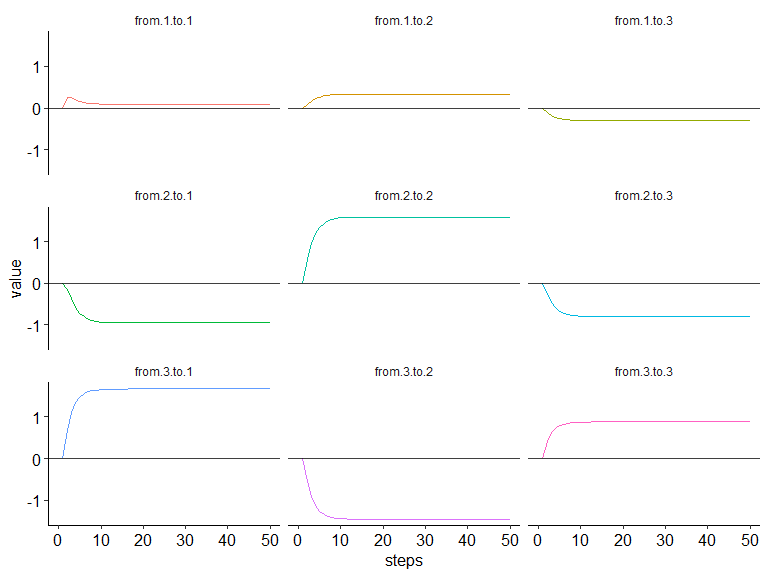## NULL